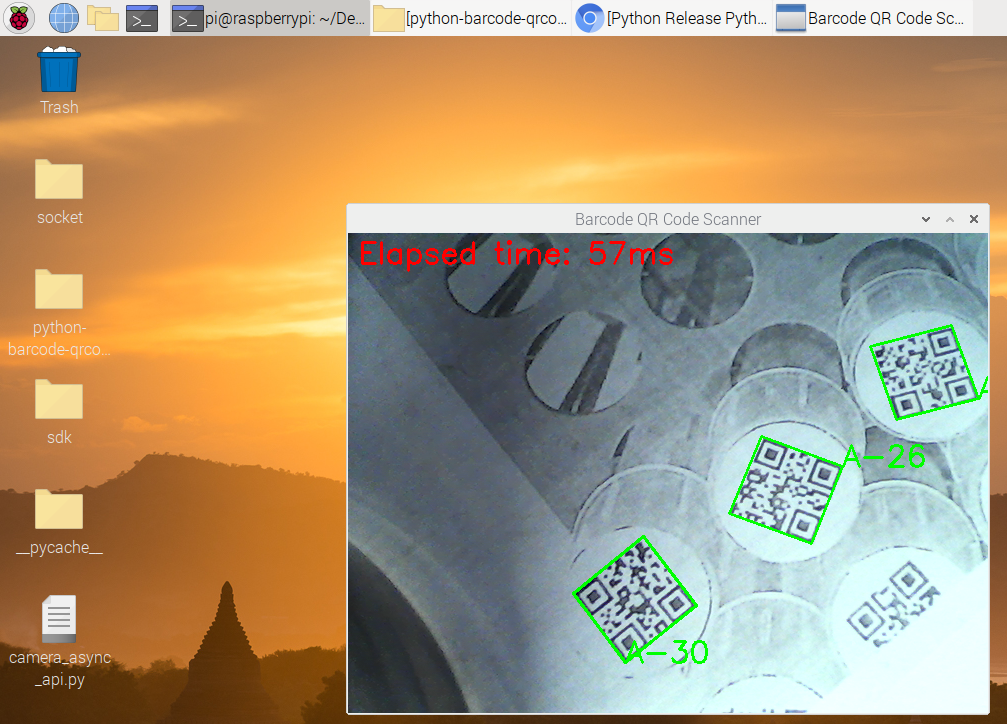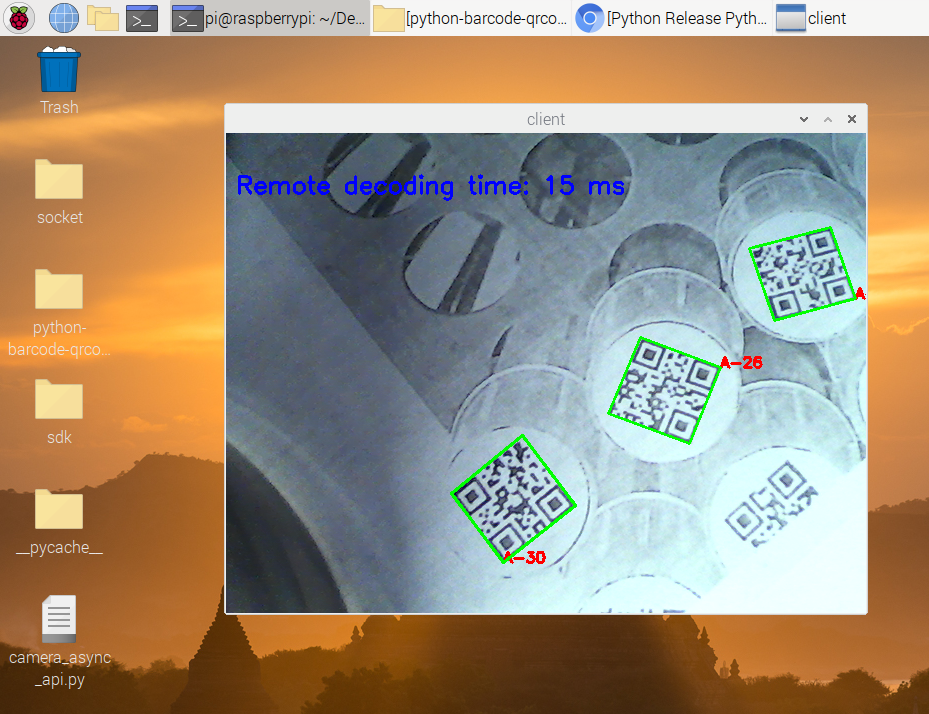×

# How to Improve Python Barcode QR Code Scanning Performance on Raspberry Pi

Raspberry Pi is an affordable single-board computer often chosen by developers for cost-effective IoT solutions, such as barcode and QR code scanning. It’s important to note that the CPU clock speed influences the decoding speed and accuracy. Dynamsoft Barcode Reader SDK, compatible with Raspberry Pi, excels in decoding multiple and low-quality barcodes, a capability that’s heavily dependent on the CPU clock rate. Even though the latest Raspberry Pi model boasts a 1.5GHz quad-core CPU, striking a balance between speed and accuracy by adjusting algorithm parameters remains challenging. In this article, I’ll guide you through scanning barcodes and QR codes asynchronously in Python on Raspberry Pi and offer insights into overcoming the CPU limitations using server-side decoding via socket.

## Prerequisites

• Python 3.6 or above
• Python Package
• `pip install dbr`: the official Python package of Dynamsoft Barcode Reader, which provides full API and relevant documentation.
• `pip install barcode-qr-code-sdk`: a community version based on Dynamsoft C/C++ Barcode SDK, providing async decoding API for easy usage.

## Building Python Barcode Scanner on Raspberry Pi

Implementing a barcode scanner in Python involves the following steps:

1. Use OpenCV to capture the video stream from the camera.
2. Use Dynamsoft Barcode Reader to decode the barcode from the image.

Barcode reading is a CPU-intensive task. When running synchronous API on a high clock rate CPU, we may not be aware of the latency on desktop computers. However, the CPU clock rate of Raspberry Pi is much lower. To avoid FPS (frames per second) dropping, it is necessary to carry out barcode detection algorithm in a separate thread.

Python’s GIL (Global Interpreter Lock) limits the thread concurrency performance, especially for CPU-intensive tasks. The `multiprocessing` module is a better choice for the barcode scanning scenario. However, it is not easy to share the memory between processes. The Python package released by Dynamsoft Barcode Reader SDK provides three asynchronous decoding methods start_video_mode(), append_video_frame and stop_video_mode to overcome the GIL limitation. They maintains a C/C++ thread pool and a buffer queue.

To simplify the native-threaded API, the community version adds an alternative method called `decodeMatAsync()`, which decodes the latest image buffer and send decoding results via a registered callback function.

``````import barcodeQrSDK
import numpy as np
import cv2
import json

g_results = None

def callback(results, elapsed_time):
global g_results
g_results = (results, elapsed_time)

def run():

# initialize barcode scanner
scanner = barcodeQrSDK.createInstance()
params = scanner.getParameters()

# register callback function to native thread

cap = cv2.VideoCapture(0)
while True:
if image is not None:
scanner.decodeMatAsync(image)

if g_results != None:
print('Elapsed time: ' + str(g_results) + 'ms')
cv2.putText(image, 'Elapsed time: ' + str(g_results) + 'ms', (10, 30), cv2.FONT_HERSHEY_SIMPLEX, 1, (0, 0, 255), 2)
for result in g_results:
x1 = result.x1
y1 = result.y1
x2 = result.x2
y2 = result.y2
x3 = result.x3
y3 = result.y3
x4 = result.x4
y4 = result.y4

cv2.drawContours(image, [np.int0([(x1, y1), (x2, y2), (x3, y3), (x4, y4)])], 0, (0, 255, 0), 2)
cv2.putText(image, result.text, (x1, y1), cv2.FONT_HERSHEY_SIMPLEX, 1, (0, 255, 0), 2)

cv2.imshow('Barcode QR Code Scanner', image)
ch = cv2.waitKey(1)
if ch == 27:
break

scanner.clearAsyncListener()

if __name__ == '__main__':
run()
``````## Moving Barcode Detection Work to Server Side

The performance of running above Python barcode scanner on Raspberry Pi 4 looks not bad. However, if you want to run the program on some older Raspberry Pi models or other cheaper single-board computers with lower CPU clock rate, the performance will be much worse. To relieve the CPU burden, we can move the heavy computation to a powerful server. Here we use Python socket programming. You can get started with the article - Socket Programming in Python.

### How to Compress Camera Frames for Socket Transmission

WebP is a modern image format that provides superior lossless and lossy compression for images. It is supported by OpenCV. The following code shows how to encode and decode an image with WebP using OpenCV API:

``````import cv2 as cv
import numpy as np

cap = cv.VideoCapture(0)
# Send
webp = cv.imencode('.webp', frame, [cv.IMWRITE_WEBP_QUALITY, 90])
bytes_sent = webp.tobytes()

``````

### A Simple Socket Class for Sending and Receiving Data

We create a `SimpleSocket` class with `socket` and `selector` modules. The `selector` is used to implement non-blocking I/O.

``````import socket
import selectors

class SimpleSocket():
def __init__(self) -> None:
self.sel = selectors.DefaultSelector()
self.sock = socket.socket(socket.AF_INET, socket.SOCK_STREAM)
self.callback = None

def registerEventCb(self, callback):
self.callback = callback

self.sock.setblocking(False)
self.sel.register(self.sock, events, data=self.callback)

def startServer(self, port, number_of_clients=1):
self.sock.bind(('', port))
self.sock.listen(number_of_clients)
print('waiting for a connection at port %s' % port)
self.sock.setblocking(False)
``````

The callback parameter is a tuple of read and write callback functions.

In an infinite loop, we call `select()` to wait for I/O events. It monitors connection, read, and write events.

``````def monitorEvents(self):
events = self.sel.select(timeout=None)
if key.data is None:
self.acceptConn(key.fileobj, self.callback)
else:

def acceptConn(self, sock, callback):
connection.setblocking(False)
self.sel.register(connection, events, data=callback)

sock = key.fileobj
callback = key.data
if callback != None and len(callback) == 2:
callback(data_type, data)
data_type, data = callback()
if data_type != None and data != None:
self.sendData(sock, data_type, data)
``````

For C/S communication, we need to define a simple protocol.

``````'''
+-+-+-+-+-------+-+-------------+-------------------------------+
|Type (1 byte)                  |   Payload length (4 bytes)    |
|0: text, 1: json 2: webp       |                               |
+-------------------------------+-------------------------------+
+-------------------------------- - - - - - - - - - - - - - - - +
:                     Payload Data continued ...                :
+ - - - - - - - - - - - - - - - - - - - - - - - - - - - - - - - +
|                     Payload Data continued ...                |
+---------------------------------------------------------------+
'''
``````

The first byte is the data type that represents text, json, or webp. The next 4 bytes are the payload length. The rest of the data is the payload data.

According to the protocol, we can implement the `receiveData()` and `sendData()` functions.

``````def sendData(self, sock, data_type, data):
msg = data_type + len(data).to_bytes(4, 'big') + data
return self.send(sock, msg)

if data_type == b'':
return b'', b''
if data_length == b'':
return b'', b''
return data_type, data

def send(self, sock, msg):
try:
totalsent = 0
while totalsent < len(msg):
sent = sock.send(msg[totalsent:])
if sent == 0:
# connection closed
return False

totalsent = totalsent + sent
except Exception as e:
print(e)
return False

return True

try:
chunks = []
bytes_recd = 0
while bytes_recd < size:
chunk = sock.recv(min(size, 1024))
if chunk == b'':
# connection closed
return b''

chunks.append(chunk)
bytes_recd = bytes_recd + len(chunk)

except Exception as e:
print(e)
return b''

return b''.join(chunks)
``````

### Implementing Server-side Barcode Scanning Solution

The steps to implement the client.

1. Create a `client.py` file.
2. Set camera resolution to 640x480 and create a loop to capture frames.
`````` import cv2 as cv
from simplesocket import SimpleSocket, DataType
import json
import numpy as np

g_local_results = None
g_remote_results = None
isDisconnected = False
msgQueue = []

cap = cv.VideoCapture(0)
if cap.isOpened() == False:
exit()

cap.set(cv.CAP_PROP_FRAME_WIDTH, 640)
cap.set(cv.CAP_PROP_FRAME_HEIGHT, 480)

def run():
while True:
cv.imshow('client', frame)
if cv.waitKey(10) == 27:
break

if __name__ == '__main__':
run()
``````
3. Initialize the socket client and register the callback functions.
`````` def callback(results, elapsed_time):
global g_local_results
print("Local decoding time: " + str(elapsed_time) + " ms")
g_local_results = (results, elapsed_time)

if data == b'':
isDisconnected = True

if data_type == DataType.TEXT:
text = data.decode('utf-8')
print(text)

if data_type == DataType.JSON:
g_remote_results = (obj['results'], obj['time'])

# Data for sending
def writeCb():
if len(msgQueue) > 0:
data_type, data =  msgQueue.pop(0)
return data_type, data

return None, None

def run():

client = SimpleSocket()
client.startClient('192.168.8.72', 8080)
``````
4. Keep reading frames from the camera and send them to the server.
`````` while True:
client.monitorEvents()
if (isDisconnected):
break

# Send data to server
webp = cv.imencode('.webp', frame, [cv.IMWRITE_WEBP_QUALITY, 90])
msgQueue.append((DataType.WEBP, webp.tobytes()))

cv.imshow('client', frame)
if cv.waitKey(10) == 27:
break

``````
5. Display the results when the results are returned from the server.
`````` if g_remote_results != None:
print("Remote decoding time: " + str(int(g_remote_results)) + " ms")
for result in g_remote_results:
text = result['text']
x1 = result['x1']
y1 = result['y1']
x2 = result['x2']
y2 = result['y2']
x3 = result['x3']
y3 = result['y3']
x4 = result['x4']
y4 = result['y4']
cv.putText(frame, text, (x1, y1), cv.FONT_HERSHEY_SIMPLEX, 0.5, (0, 0, 255), 2)
cv.drawContours(frame, [np.int0([(x1, y1), (x2, y2), (x3, y3), (x4, y4)])], 0, (0, 255, 0), 2)

cv.putText(frame, "Remote decoding time: " + str(int(g_remote_results)) + " ms", (10, 60), cv.FONT_HERSHEY_SIMPLEX, 0.8, (255, 0, 0), 2)
``````

Considering the CPU performance is good and there is no UI required on the server side, we can use synchronous API to recognize barcode and Qr code after receiving the frame from the client. The returned results are encoded as JSON string.

``````import cv2 as cv
import numpy as np
from simplesocket import SimpleSocket, DataType
import json
import barcodeQrSDK

g_results = None
isDisconnected = False
msgQueue = []

global isDisconnected, g_results, msgQueue

if data == b'':
isDisconnected = True

if data_type == DataType.TEXT:
text = data.decode('utf-8')
print(text)

if data_type == DataType.JSON:
print(obj)

if data_type == DataType.WEBP:
try:

if frame is not None:
g_results = (results, elpased_time)

if g_results != None:
jsonData = {'results': [], 'time': g_results}
for result in g_results:
format = result.format
text = result.text
x1 = result.x1
y1 = result.y1
x2 = result.x2
y2 = result.y2
x3 = result.x3
y3 = result.y3
x4 = result.x4
y4 = result.y4
data = {'format': format, 'text': text, 'x1': x1, 'y1': y1, 'x2': x2, 'y2': y2, 'x3': x3, 'y3': y3, 'x4': x4, 'y4': y4}
jsonData['results'].append(data)

msgQueue.append((DataType.JSON, json.dumps(jsonData).encode('utf-8')))

except Exception as e:
isDisconnected = True

def writeCb():
if len(msgQueue) > 0:
data_type, data =  msgQueue.pop(0)
return data_type, data

return None, None

def run():
global isDisconnected

server = SimpleSocket()
server.startServer(8080, 1)

try:
while True:
server.monitorEvents()
if (isDisconnected):
break

except KeyboardInterrupt:
print("Caught keyboard interrupt, exiting")
finally:
server.shutdown()

if __name__ == '__main__':
run()

``````

### Testing the Server-side Barcode Scanning Solution

When running the `client.py` and `server.py`, you need to change the IP address and port. If the network transmission is stable, the server-side barcode scanning solution can achieve good performance.## Source Code

https://github.com/yushulx/python-barcode-qrcode-sdk/tree/main/examples/socket